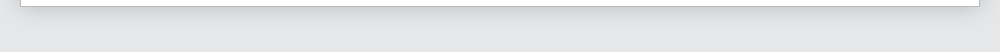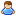Login Products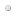SalesSupportDownloadsAbout
 Home » Technical Support » Elevate Web Builder Technical Support » Product Manuals » Elevate Web Builder 3 Manual » Language Reference » Operators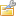Operators

Elevate Web Builder supports most Object Pascal operators, and these operators are detailed below.

Boolean Operators
The following are the boolean operators, ordered by their operator precedence:

 Operator Description
 not Flips a boolean expression so that True becomes False, or vice-versa. and Returns True if both the left and right boolean expressions are True. or Returns True if either the left or right boolean expression is True.

Comparison Operators
The following are the comparison operators, ordered by their operator precedence:

 Operator Description
 = Returns True if both the left and right expressions are equal. <> Returns True if both the left and right expressions are not equal. > Returns True if the left expression is greater than the right expression. >= Returns True if the left expression is greater than or equal to the right expression. < Returns True if the left expression is less than the right expression. <= Returns True if the left expression is less than or equal to the right expression. is Returns True if the left expression is an instance of the class type specified in the right expression.

Arithmetic Operators
The following are the arithmetic operators, ordered by their operator precedence:

 Operator Description
 not Returns an integer that represents the inverse of all bits in the right integer expression. or Returns an integer that represents all set bits in the left and right integer expressions. xor Returns an integer that represents all set bits in either the left or right, but not both, integer expressions. and Returns an integer that represents all bits that are set in both the left and right integer expressions. * Multiplies the left numeric expression by the right numeric expression. / Divides the left numeric expression by the right numeric expression. div Divides the left integer expression by the right integer expression. - Subtracts the right numeric expression from the left numeric expression. + Adds the right numeric expression to the left numeric expression. mod Returns the remainder derived from dividing the left numeric expression by the right numeric expression. shl Returns the left integer expression shifted to the left by the number of bits specified by the right integer expression. shr Returns the left integer expression shifted to the right by the number of bits specified by the right integer expression.

String Operators
The following are the string operators, ordered by their operator precedence:

 Operator Description
 + Concatenates the right string expression to the left string expression.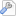More Support Options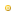Support ForumsFrequently Asked QuestionsTechnical BulletinsTechnical ArticlesIncident ReportsRoadmap